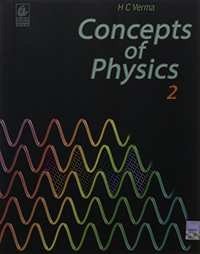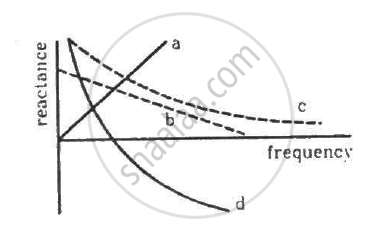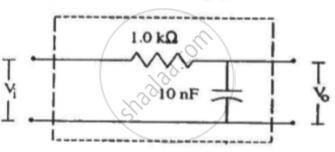CBSE (Science) Class 11CBSE
Share

# HC Verma solutions for Class 11 Physics chapter 17 - Alternating Current

#### HC Verma Concepts of Physics - Vol. 2## Chapter 17: Alternating Current

#### HC Verma solutions for Class 11 Physics Chapter 17 Exercise Short Answers [Pages 328 - 329]

Short Answers | Q 1 | Page 328

What is the reactance of a capacitor connected to a constant DC source?

Short Answers | Q 2 | Page 328

The voltage and current in a series AC circuit are given by V = V0cos ωt and i = i0 sin ωt. What is the power dissipated in the circuit?

Short Answers | Q 3 | Page 328

Two alternating currents are given by i_1 = i_0 sin wt and i_2 = i_0 sin (wt + pi/3) Will the rms values of the currents be equal or different?

Short Answers | Q 4 | Page 328

Can the peak voltage across the inductor be greater than the peak voltage of the source in an LCR circuit?

Short Answers | Q 5 | Page 328

In a circuit, containing a capacitor and an AC source, the current is zero at the instant the source voltage is maximum. Is it consistent with Ohm's Law?

Short Answers | Q 6 | Page 328

An AC source is connected to a capacitor. Will the rms current increase, decrease or remain constant if a dielectric slab is inserted into the capacitor?

Short Answers | Q 7 | Page 328

When the frequency of the AC source in an LCR circuit equals the resonant frequency, the reactance of the circuit is zero. Does it mean that there is no current through the inductor or the capacitor?

Short Answers | Q 8 | Page 328

When an AC source is connected to a capacitor, there is a steady-state current in the circuit. Does it mean that the charges jump from one plate to the other to complete the circuit?

Short Answers | Q 9 | Page 329

A current i1 = i0 sin ωt passes through a resistor of resistance R. How much thermal energy is produced in one time period? A current i2 = −i0 sin ωt passes through the resistor. How much thermal energy is produced in one time period? If i1 and i2 both pass through the resistor simultaneously, how much thermal energy is produced? Is the principle of superposition obeyed in this case?

Short Answers | Q 10 | Page 329

Is energy produced when a transformer steps up the voltage?

Short Answers | Q 11 | Page 329

A transformer is designed to convert an AC voltage of 220 V to an AC voltage of 12 V. If the input terminals are connected to a DC voltage of 220 V, the transformer usually burns. Explain.

Short Answers | Q 12 | Page 329

Can you have an AC series circuit in which there is a phase difference of (a) 180° (b) 120° between the emf and the current?

Short Answers | Q 13 | Page 329

A resistance is connected to an AC source. If a capacitor is included in the series circuit, will the average power absorbed by the resistance increase or decrease? If an inductor of small inductance is also included in the series circuit, will the average power absorbed increase or decrease further?

Short Answers | Q 14 | Page 329

Can a hot-wire ammeter be used to measure a direct current of constant value? Do we have to change the graduations?

#### HC Verma solutions for Class 11 Physics Chapter 17 Exercise MCQ [Page 329]

MCQ | Q 1 | Page 329

A capacitor acts as an infinite resistance for

•  DC

•  DC

•  DC as well as AC

• neither AC nor DC

MCQ | Q 2 | Page 329

An AC source producing emf ε = ε0 [cos (100 π s−1)t + cos (500 π s−1)t] is connected in series with a capacitor and a resistor. The steady-state current in the circuit is found to be i1 cos [(100 π s−1)t + φ1) + i2 cos [(500π s−1)t + ϕ2]. So,

• i1 > i2

• i1 = i2

•  i1 < i2

• The information is insufficient to find the relation between i1 and i2.

MCQ | Q 3 | Page 329

The peak voltage of a 220 V AC source is

• 220 V

• 440 V

MCQ | Q 4 | Page 329

An AC source is rated 220 V, 50 Hz. The average voltage is calculated in a time interval of 0.01 s. It

• must be zero

• may be zero

• is never zero

• is (220//sqrt2 )V

MCQ | Q 5 | Page 329

The magnetic field energy in an inductor changes from maximum to minimum value in 5.0 ms when connected to an AC source. The frequency of the source is

• 20 Hz

• 50 Hz

•  200 Hz

• 500 Hz

MCQ | Q 6 | Page 329

Which of the following plots may represent the reactance of a series LC combination?MCQ | Q 7 | Page 329

A series AC circuit has a resistance of 4 Ω and a reactance of 3 Ω. The impedance of the circuit is

•  5 Ω

•  7 Ω

• 12/7 Ω

• 7/12 Ω

MCQ | Q 8 | Page 329

Transformers are used

• in DC circuits only

• in AC circuits only

• in both DC and AC circuits

• neither in DC nor in AC circuits

MCQ | Q 9 | Page 329

An alternating current is given by i = i1 cos ωt + i2 sin ωt. The rms current is given by

• (l_1 +l_2)/sqrt2

• |i_1 + i_2|/sqrt2

• sqrt(i_1^2 + i_2^2)/2

• sqrt(i_1^2+i_2^2)/sqrt2

MCQ | Q 10 | Page 329

An alternating current of peak value 14 A is used to heat a metal wire. To produce the same heating effect, a constant current i can be used, where i is

• 14 A

•  7 A

MCQ | Q 11 | Page 329

A constant current of 2.8 A exists in a resistor. The rms current is

• 2.8 A

•  1.4 A

•  undefined for a direct current

#### HC Verma solutions for Class 11 Physics Chapter 17 Exercise MCQ [Pages 329 - 330]

MCQ | Q 1 | Page 329

An inductor, a resistance and a capacitor are joined in series with an AC source. As the frequency of the source is slightly increased from a very low value, the reactance

• of the inductor increases

• of the resistor increases

•  of the capacitor increases

• of the circuit increases

MCQ | Q 2 | Page 329

The reactance of a circuit is zero. It is possible that the circuit contains
(a) an inductor and a capacitor
(b) an inductor but no capacitor
(c) a capacitor but no inductor
(d) neither an inductor nor a capacitor

MCQ | Q 3 | Page 329

In an AC series circuit, the instantaneous currt is zero when the instantaneous voltage is maximum. Connected to the source may be a
(a) pure inductor
(b) pure capacitor
(c) pure resistor
(d) combination of an inductor and a capacitor

MCQ | Q 4 | Page 329

An inductor coil of some resistance is connected to an AC source. Which of the following quantities have zero average value over a cycle?
(a) Current
(b) Induced emf in the inductor
(c) Joule heat
(d) Magnetic energy stored in  the inductor

MCQ | Q 5 | Page 330

The AC voltage across a resistance can be measured using

• a potentiometer

• a hot-wire voltmeter

• a moving-magnet galvanometer

MCQ | Q 6 | Page 330

To convert mechanical energy into electrical energy, one can use

(a) DC dynamo
(b) AC dynamo
(c) motor
(d) transformer

MCQ | Q 7 | Page 330

An AC source rated 100 V (rms) supplies a current of 10 A (rms) to a circuit. The average power delivered by the source
(a) must be 1000 W
(b) may be 1000 W
(c) may be greater than 1000 W
(d) may be less than 1000 W

#### HC Verma solutions for Class 11 Physics Chapter 17 [Pages 33 - 331]

Q 1 | Page 330

Find the time required for a 50 Hz alternating current to change its value from zero to the rms value.

Q 2 | Page 330

The household supply of electricity is at 220 V (rms value) and 50 Hz. Find the peak voltage and the least possible time in which the voltage can change from the rms value to zero.

Q 3 | Page 330

A bulb rated 60 W at 220 V is connected across a household supply of alternating voltage of 220 V. Calculate the maximum instantaneous current through the filament.

Q 4 | Page 330

An electric bulb is designed to operate at 12 volts DC. If this bulb is connected to an AC source and gives normal brightness, what would be the peak voltage of the source?

Q 5 | Page 330

The peak power consumed by a resistive coil, when connected to an AC source, is 80 W. Find the energy consumed by the coil in 100 seconds, which is many times larger than the time period of the source.

Q 6 | Page 330

The dielectric strength of air is 3.0 × 106 V/m. A parallel-plate air-capacitor has area 20 cm2 and plate separation 0.10 mm. Find the maximum rms voltage of an AC source that can be safely connected to this capacitor.

Q 7 | Page 330

The current in a discharging LR circuit is given by i = i0 et , where τ is the time constant of the circuit. Calculate the rms current for the period = 0 to t = τ.

Q 8 | Page 33

A capacitor of capacitance 10 μF is connected to an oscillator with output voltage ε = (10 V) sin ωt. Find the peak currents in the circuit for ω = 10 s−1, 100 s−1, 500 s−1 and 1000 s−1.

Q 9 | Page 330

A coil of inductance 5.0 mH and negligible resistance is connected to the oscillator of the previous problem. Find the peak currents in the circuit for ω = 100 s−1, 500 s−1, 1000 s−1.

Q 10 | Page 330

A coil has a resistance of 10 Ω and an inductance of 0.4 henry. It is connected to an AC source of 6.5 V, 30/pi Hz. Find the average power consumed in the circuit.

Q 11 | Page 330

A resistor of resistance 100 Ω is connected to an AC source ε = (12 V) sin (250 π s−1)t. Find the energy dissipated as heat during t = 0 to t = 1.0 ms.

Q 12 | Page 330

In a series RC circuit with an AC source, R = 300 Ω, C = 25 μF, ε0 = 50 V and ν = 50/π Hz. Find the peak current and the average power dissipated in the circuit.

Q 13 | Page 330

An electric bulb is designed to consume 55 W when operated at 110 volts. It is connected to a 220 V, 50 Hz line through a choke coil in series. What should be the inductance of the coil for which the bulb gets correct voltage?

Q 14 | Page 330

In a series LCR circuit with an AC source, R = 300 Ω, C = 20 μF, L = 1.0 henry, εrms = 50 V and ν = 50/π Hz. Find (a) the rms current in the circuit and (b) the rms potential difference across the capacitor, the resistor and the inductor. Note that the sum of the rms potential differences across the three elements is greater than the rms voltage of the source.

Q 15 | Page 330

Consider the situation of the previous problem. Find the average electric field energy stored in the capacitor and the average magnetic field energy stored in the coil.

Q 16 | Page 330

An inductance of 2.0 H, a capacitance of 18μF and a resistance of 10 kΩ are connected to an AC source of 20 V with adjustable frequency. (a) What frequency should be chosen to maximise the current in the circuit? (b) What is the value of this maximum current?

Q 17 | Page 330

An inductor-coil, a capacitor and an AC source of rms voltage 24 V are connected in series. When the frequency of the source is varied, a maximum rms current of 6.0 A is observed. If this inductor coil is connected to a battery of emf 12 V and internal resistance 4.0 Ω, what will be the current?

Q 18 | Page 330

Following figure shows a typical circuit for a low-pass filter. An AC input V= 10 mV is applied at the left end and the output V0 is received at the right end. Find the output voltage for ν = 10 k Hz, 1.0 MHz and 10.0 MHz. Note that as the frequency is increased the output decreases and, hence, the name low-pass filter.Q 19 | Page 331

A transformer has 50 turns in the primary and 100 in the secondary. If the primary is connected to a 220 V DC supply, what will be the voltage across the secondary?

## Chapter 17: Alternating Current

#### HC Verma Concepts of Physics - Vol. 2## HC Verma solutions for Class 11 Physics chapter 17 - Alternating Current

HC Verma solutions for Class 11 Physics chapter 17 (Alternating Current) include all questions with solution and detail explanation. This will clear students doubts about any question and improve application skills while preparing for board exams. The detailed, step-by-step solutions will help you understand the concepts better and clear your confusions, if any. Shaalaa.com has the CBSE Concepts of Physics - Vol. 2 solutions in a manner that help students grasp basic concepts better and faster.

Further, we at Shaalaa.com provide such solutions so that students can prepare for written exams. HC Verma textbook solutions can be a core help for self-study and acts as a perfect self-help guidance for students.

Concepts covered in Class 11 Physics chapter 17 Alternating Current are Lc Oscillations, Reactance and Impedance, Peak and Rms Value of Alternating Current Or Voltage, Alternating Currents, Power in Ac Circuit: the Power Factor, Ac Voltage Applied to a Series Lcr Circuit, Ac Voltage Applied to an Inductor, Representation of Ac Current and Voltage by Rotating Vectors - Phasors, Ac Voltage Applied to a Resistor, Alternating Currents and Direct Currents, Forced Oscillations and Resonance, Ac Voltage Applied to a Capacitor, Transformers.

Using HC Verma Class 11 solutions Alternating Current exercise by students are an easy way to prepare for the exams, as they involve solutions arranged chapter-wise also page wise. The questions involved in HC Verma Solutions are important questions that can be asked in the final exam. Maximum students of CBSE Class 11 prefer HC Verma Textbook Solutions to score more in exam.

Get the free view of chapter 17 Alternating Current Class 11 extra questions for Physics and can use Shaalaa.com to keep it handy for your exam preparation

S